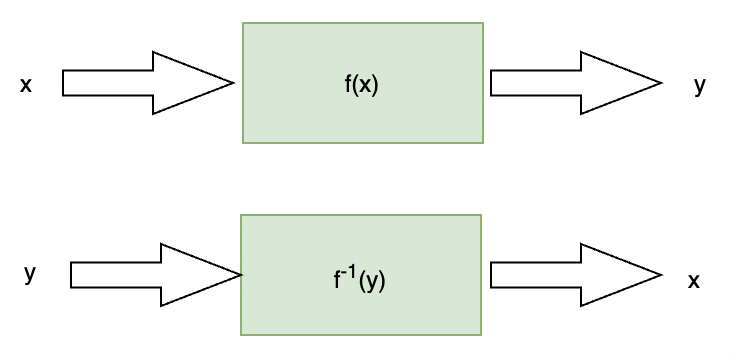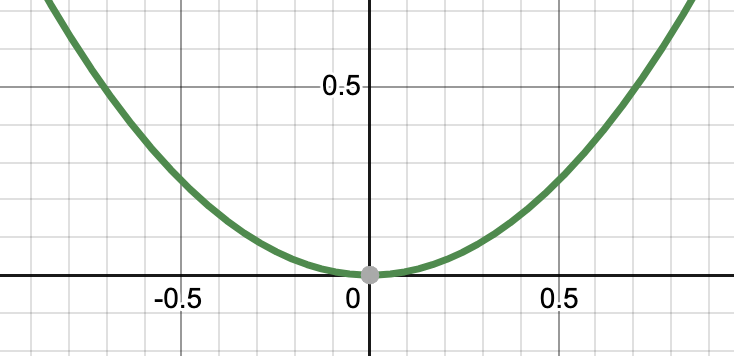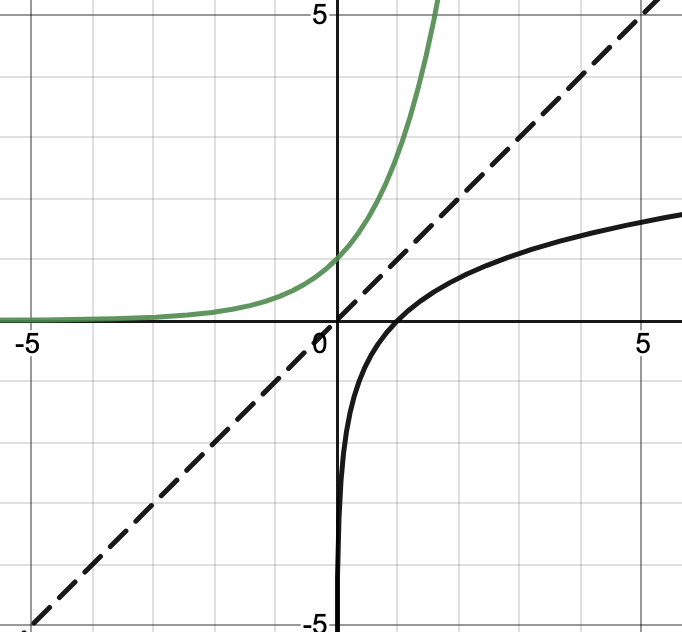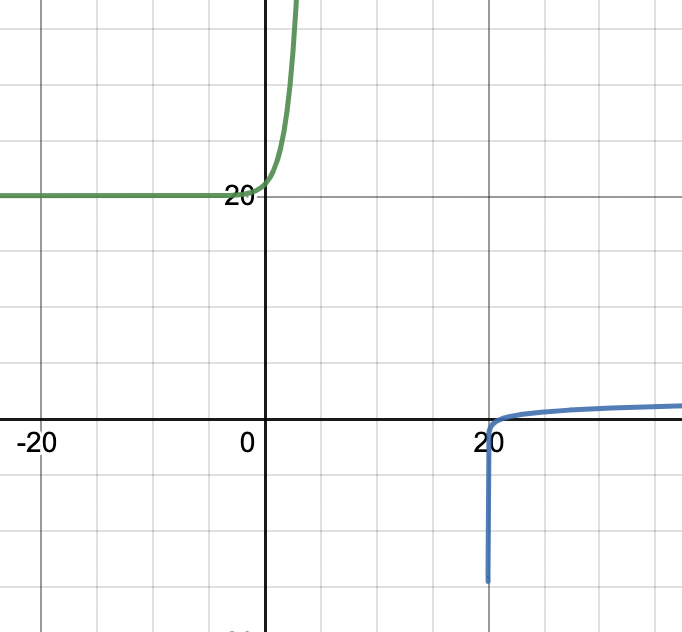Open in App
Not now

# Inverse Functions

• Last Updated : 30 Apr, 2021

We know that the functions are just like a machine or a box which takes an input value and gives us some output value after processing. There should be only one output corresponding to one input. Sometimes it can happen that we have the output, and we want to know the input. In such cases, we need to revert whatever the processing was applied on input. So it becomes essential to know the reverse of the process. If that function is mathematical we need to know what is the inverse of the function so that we can get back our input. Let’s see inverses and the process in detail.

### Introduction to Inverse Functions

The figure given below describes a function and its inverse. This function is represented as f(x) and takes some input values and gives an output. The inverse of this function is denoted by f-1(x). For example, let’s say f(x) = 2x. It doubles the number which is given as input, its inverse should make them half to get back the input. f-1(x) =If functions f(x) and g(x) are inverses of each other, then f(x) = y only if g(y) = x.

g(f(x)) = x

Let’s say we have a function f(x) = x2. Now we are asked to find out the inverse of this function. This function is squaring its inputs, we know we need to take the square root for calculating the inverse.

f-1(x) = √x2

= ±x

We see that there are two answers possible, which one to choose? In such cases, the inverse is not possible. So, there are things we need to notice for the functions for which inverses are possible.

• Function must be Bijective(One-One and Onto) for its inverse to exist.

This function has same values at two different values of input. This function is not ideal for calculating inverse.• We need to look for the points which are not in domain for the inverse. For example, in the previous case, negative values cannot be allowed.

### Inverses with Algebraic Methods

The inverses of functions can also be calculated using algebraic methods. The idea is, to substitute the value of y in place of f(x) and then solve for y. Let’s see an example to understand it.

Example: Find the inverse of f(x) = 6x + 10.

Solution:

We know, f(x) = 6x + 10. Let’s substitute y in place of f(x).

y = 6x + 10

⇒ y – 10 = 6x

⇒ x =f-1(y) =### Inverses of Common Functions

The table given below describes inverses of some common functions which may come in handy while calculating the inverses for complex functions. The table represents the function, its inverse, and its corner cases. Corner cases describe the values which are not allowed as input to the inverse of the function.

### Graphs of Inverse Functions

We will see the shape of the graph of the inverse of a function through an example. Let’s say we have f(x) = ex. Let’s say the inverse of this function is g(x), we know that the inverse of an exponential function is a logarithmic function. So, g(x) = logex. The figure below shows the graph for both of the functions.Notice in the figure, you can see that both the curves are mirror images of each other with respect to the line y = x. So, we can say that the inverse of a function is a mirror image of the function when seen through the line y = x.

Let’s see some problems on these concepts.

### Sample Problems

Question 1: Find the inverse of the function f(x) =Solution:Substituting f(x) with y.⇒⇒ 2xy + y = x + 4

⇒ x(2y – 1) = 4 – y

⇒ x =Thus, f-1(y) =Question 2: Find the inverse of the function f(x) = lnx + 5.

Solution:

f(x) = lnx + 5

Substituting the f(x) with y

y = lnx + 5

⇒ lnx= y – 5

⇒ x = e(y – 5)

f-1(y) = e(y – 5)

Question 3: Find the inverse of the following function and draw its graph.

f(x) = ex + 20

Solution:

f(x) = ex + 20

Substituting the f(x) with y

⇒y = ex + 20

⇒y – 20 = ex

⇒ln(y – 20) = x

f-1(y) = ln(y – 20)

The figure below, shows the graphs for f(x) and it’s inverse.Notice that y > 20 for this function.

Question 4: State whether the statement is True or False. For the given function f(x) = x2 + 4, inverse does not exist for all values of x.

Solution:

We know that f(x) = x2 + 4 is not bijective. For example,

f(-2) = 8 and f(2) = 8. So, the inverse for this function cannot exist for all values of x. Thus, this statement is called False.

Question 5: Find the inverse for the following function:

f(x) =Solution:

f(x) =Substituting f(x) with y.⇒⇒ y(5x + 1) = x

⇒ 5xy + y = x

⇒ x(5y – 1) = -y

⇒ x =Thus, f-1(y) =My Personal Notes arrow_drop_up